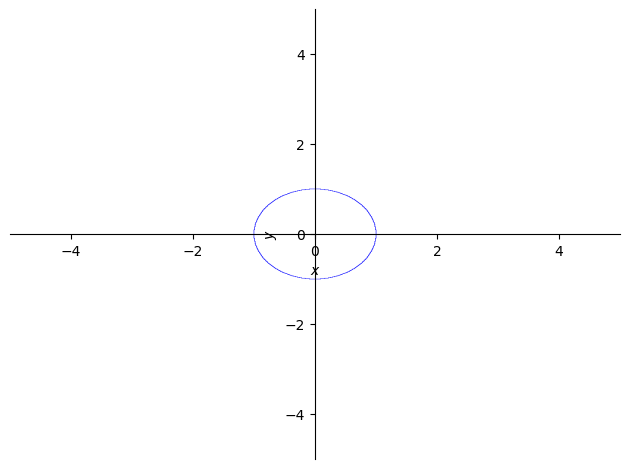# How to graph curves that are not functions

## Description

Assume we have an equation in which $y$ cannot be isolated as a function of $x$. (The standard example is the formula for the unit circle, $x^2+y^2=1$.) We would still like to be able to use software to plot such curves. How?

## Using SymPy, in Python

View this solution alone.

This answer assumes you have imported SymPy as follows.

1
2
from sympy import *                   # load all math functions
init_printing( use_latex='mathjax' )  # use pretty math output


Let’s consider the example of the unit circle, $x^2+y^2=1$.

To plot it, SymPy first expects us to move everything to the left-hand side of the equation, so in this case, we would have $x^2+y^2-1=0$.

We then use that left hand side to represent the equation as a single formula, and we can plot it with SymPy’s plot_implicit function.

1
2
3
var( 'x y' )
formula = x**2 + y**2 - 1   # to represent x^2+y^2=1
plot_implicit( formula )1
<sympy.plotting.plot.Plot at 0x7f67130cb460>


See a problem? Tell us or edit the source.

## Opportunities

This website does not yet contain a solution for this task in any of the following software packages.

• R
• Excel
• Julia

If you can contribute a solution using any of these pieces of software, see our Contributing page for how to help extend this website.